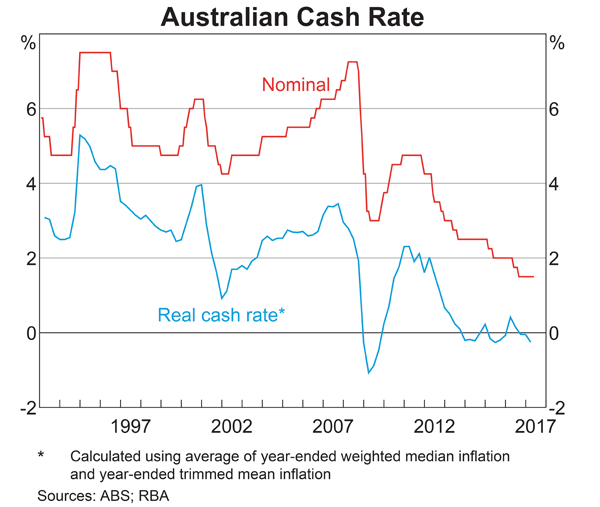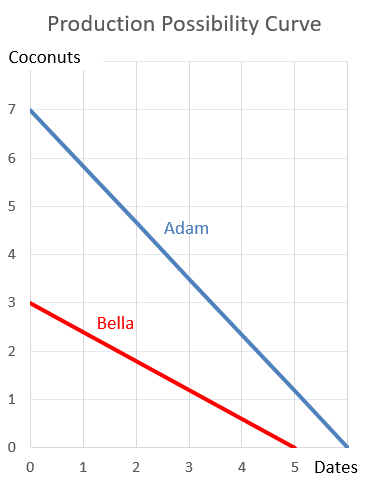# Fight Finance

#### CoursesTagsRandomAllRecentScores

Bonds X and Y are issued by the same US company. Both bonds yield 10% pa, and they have the same face value ($100), maturity, seniority, and payment frequency. The only difference is that bond X and Y's coupon rates are 8 and 12% pa respectively. Which of the following statements is true? In the dividend discount model: $$P_0= \frac{d_1}{r-g}$$ The pronumeral $g$ is supposed to be the: A risky firm will last for one period only (t=0 to 1), then it will be liquidated. So it's assets will be sold and the debt holders and equity holders will be paid out in that order. The firm has the following quantities: $V$ = Market value of assets. $E$ = Market value of (levered) equity. $D$ = Market value of zero coupon bonds. $F_1$ = Total face value of zero coupon bonds which is promised to be paid in one year. What is the payoff to debt holders at maturity, assuming that they keep their debt until maturity? A stock just paid a dividend of$1. Future annual dividends are expected to grow by 2% pa. The next dividend of $1.02 (=1*(1+0.02)^1) will be in one year, and the year after that the dividend will be$1.0404 (=1*(1+0.02)^2), and so on forever.

Its required total return is 10% pa. The total required return and growth rate of dividends are given as effective annual rates.

Calculate the current stock price.

The below table summarises the borrowing costs confronting two companies A and B.

 Bond Market Yields Fixed Yield to Maturity (%pa) Floating Yield (%pa) Firm A 3 L - 0.4 Firm B 5 L + 1

Firm A wishes to borrow at a floating rate and Firm B wishes to borrow at a fixed rate. Design an intermediated swap (which means there will actually be two swaps) that nets a bank 0.1% and shares the remaining swap benefits between Firms A and B equally. Which of the following statements about the swap is NOT correct?

An equity index fund manager controls a USD500 million diversified equity portfolio with a beta of 0.9. The equity manager expects a significant rally in equity prices next year. The market does not think that this will happen. If the fund manager wishes to increase his portfolio beta to 1.5, how many S&P500 futures should he buy?

The US market equity index is the S&P500. One year CME futures on the S&P500 currently trade at 2,155 points and the spot price is 2,180 points. Each point is worth $250. The number of one year S&P500 futures contracts that the fund manager should buy is: Which of the following statements about an option (either a call or put) and its underlying stock is NOT correct?  European Call Option on a non-dividend paying stock Description Symbol Quantity Spot price ($) $S_0$ 20 Strike price ($) $K_T$ 18 Risk free cont. comp. rate (pa) $r$ 0.05 Standard deviation of the stock's cont. comp. returns (pa) $\sigma$ 0.3 Option maturity (years) $T$ 1 Call option price ($) $c_0$ 3.939488 Delta $\Delta = N[d_1]$ 0.747891 $N[d_2]$ $N[d_2]$ 0.643514 Gamma $\Gamma$ 0.053199 Theta ($/year) $\Theta = \partial c / \partial T$ 1.566433 Below is the Australian central bank’s cash rate.From 2011 to 2017 the Australian central bank has implemented: Adam and Bella are the only people on a remote island. Their production possibility curves are shown in the graph.Which of the following statements is NOT correct? A bank sells a European call option on a non-dividend paying stock and delta hedges on a daily basis. Below is the result of their hedging, with columns representing consecutive days. Assume that there are 365 days per year and interest is paid daily in arrears.  Delta Hedging a Short Call using Stocks and Debt Description Symbol Days to maturity (T in days) 60 59 58 57 56 55 Spot price ($) S 10000 10125 9800 9675 10000 10000 Strike price ($) K 10000 10000 10000 10000 10000 10000 Risk free cont. comp. rate (pa) r 0.05 0.05 0.05 0.05 0.05 0.05 Standard deviation of the stock's cont. comp. returns (pa) σ 0.4 0.4 0.4 0.4 0.4 0.4 Option maturity (years) T 0.164384 0.161644 0.158904 0.156164 0.153425 0.150685 Delta N[d1] = dc/dS 0.552416 0.582351 0.501138 0.467885 0.550649 0.550197 Probability that S > K at maturity in risk neutral world N[d2] 0.487871 0.51878 0.437781 0.405685 0.488282 0.488387 Call option price ($) c 685.391158 750.26411 567.990995 501.487157 660.982878 ? Stock investment value ($) N[d1]*S 5524.164129 5896.301781 4911.152036 4526.788065 5506.488143 ? Borrowing which partly funds stock investment ($) N[d2]*K/e^(r*T) 4838.772971 5146.037671 4343.161041 4025.300909 4845.505265 ? Interest expense from borrowing paid in arrears ($) r*N[d2]*K/e^(r*T) 0.662891 0.704985 0.594994 0.551449 ? Gain on stock ($) N[d1]*(SNew - SOld) 69.052052 -189.264008 -62.642245 152.062648 ? Gain on short call option ($) -1*(cNew - cOld) -64.872952 182.273114 66.503839 -159.495721 ? Net gain ($) Gains - InterestExpense 3.516209 -7.695878 3.266599 -7.984522 ? Gamma Γ = d^2c/dS^2 0.000244 0.00024 0.000255 0.00026 0.000253 0.000255 Theta θ = dc/dT 2196.873429 2227.881353 2182.174706 2151.539751 2266.589184 2285.1895

In the last column when there are 55 days left to maturity there are missing values. Which of the following statements about those missing values is NOT correct?